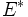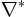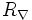# Difference between revisions of "Dual connection to flat connection is flat"

## Statement

### Statement in the language of connections

Suppose$M$ is a differential manifold,$E$ is a vector bundle over$M$, and$\nabla$ is a connection on$M$. Suppose$E^*$ is the dual bundle to$E$ and$\nabla^*$ is the dual connection to$\nabla$.

Then, if$\nabla$ is a flat connection (i.e., the Riemann curvature tensor$R_\nabla$ is identically zero),$\nabla^*$ is also a flat connection.

### Statement in the language of modules over the connection algebra

Fill this in later

## Facts used

1. Formula for curvature of dual connection